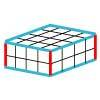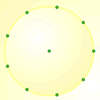#### You may also like### Round and Round the Circle

What happens if you join every second point on this circle? How about every third point? Try with different steps and see if you can predict what will happen.### Making Cuboids

Let's say you can only use two different lengths - 2 units and 4 units. Using just these 2 lengths as the edges how many different cuboids can you make?How many DIFFERENT quadrilaterals can be made by joining the dots on the 8-point circle?

# Pumpkin Pie Problem

##### Age 7 to 11Challenge Level

It looks like Peter is going to have to go shopping!

A way to think about this problem was to find out what proportion of the original recipe Peter required to make the two pies. You might have divided and then perhaps multiplied, depending on your strategy. Using what you know about fractions could have helped. A number of you sent the answer that Peter was short of some of the ingredients. There were two ways that the problem was solved and fully explained. These mathematicians came up with the following solutions and used their knowledge of decimals.

Ruth who lives in Gloustershire, decided that the answer to the Pumpkin Pie Problem is, he hasn't got enough ingredients to make two pies. She got the answer by dividing the ingredients by 80 and multipliyingby 2. Both Helena from Tattingstone School, and George of Rosebank Primary School, Leeds, divided mother's recipe by 40. Why do you think they decided to do this?

Here's Ruth's solution, she did an excellent job of setting out her calculations in a chart.

80 pies take What he needs What he has got
120 Eggs 3 eggs 2 eggs
27L Milk 0.675L Milk 0.66L Milk
480 tbs. Sugar 12 tbs. Sugar 15 tbs. Sugar
100 teasp. Cinnamon 2.5 teasp Cinnamon 1.5 teasp Cinnamon
140 cups of Pumpkin 3.5 cups of Pumpkin 4 cups of Pumpkin

Helena and George both provided clear explanations. George explained: "To find out if Peter had enough to make 2 pies I divided each of the ingredients by 40. For the 10 dozen eggs I first multiplied 10 by 12, because dozen means 12. Then I divided the answer by 40.
10 X12 = 120.

120 / 40 = 12 / 4 = 3

Peter had 2 eggs but he needed 3 eggs so he had to buy 1 more egg.

To find out how many litres of condensed milk was needed, I first converted the litres of condensed milk into millilitres of condensed milk. Then I divided the answer by 40 again.
27litres X 1000 = 27,000ml

27,000ml / 40 = 675ml

Peter had 666ml but he needed 675ml so he needed to buy 9ml of condensed milk.

To find out how many tablespoons of sugar Peter needed I just divided the amount for 80 pies by 40.
480 / 40 = 12 tablespoons of sugar.